Springer Nature is making SARS-CoV-2 and COVID-19 research free. View research | View latest news | Sign up for updates

# Global Existence of Compressible Dissipative Elastodynamics Systems with Zero Shear Viscosity in Two Dimensions

• 315 Accesses

• 2 Citations

## Abstract

In this paper, we study the two dimensional compressible dissipative elastodynamics system with zero shear viscosity, and prove global stability around a constant equilibrium. Due to the low pointwise dispersive estimate, $$\frac{1}{\sqrt{t}}$$, for the divergence-free projection of solutions in dimension two, and the lack of null structures in convective terms, energy estimates, combined with Green’s functions and dispersive estimates, for both solutions and their potentials, are obtained to control the interactions in nonlinear terms.

This is a preview of subscription content, log in to check access.

## References

1. 1.

Agemi, R.: Global existence of nonlinear elastic waves. Invent. Math. 142(2), 225–250, 2000

2. 2.

Alinhac, S.: The null condition for quasilinear wave equations in two space dimensions I. Invent. Math. 145(3), 597–618, 2001

3. 3.

Caffarelli, L., Kohn, R., Nirenberg, L.: First order interpolation inequalities with weights. Compos. Math. 53(3), 259–275, 1984

4. 4.

Cai, Y., Lei, Z., Lin, F., Masmoudi, N.: Vanishing viscosity limit for incompressible viscoelasticity in two dimensions. ArXiv e-prints, 1703, 2017

5. 5.

Cai, Y., Lei, Z., Masmoudi, N.: Global well-posedness for 2D nonlinear wave equations without compact support. J. Math. Pures Appl. 9(114), 211–234, 2018

6. 6.

Christodoulou, D.: Global solutions of nonlinear hyperbolic equations for small initial data. Commun. Pure Appl. Math. 39(2), 267–282, 1986

7. 7.

Dafermos, C.M.: Hyperbolic conservation laws in continuum physics, vol. 325, 4th edn. Grundlehren der Mathematischen Wissenschaften [Fundamental Principles of Mathematical Sciences] Springer, Berlin, 2016

8. 8.

Danchin, R.: Global existence in critical spaces for compressible navier-stokes equations. Invent. Math. 141(3), 579–614, 2000

9. 9.

Hoff, D., Zumbrun, K.: Multi-dimensional diffusion waves for the Navier-Stokes equations of compressible flow. Indiana Univ. Math. J. 44(2), 603–676, 1995

10. 10.

Hu, X., Wang, D.: Local strong solution to the compressible viscoelastic flow with large data. J. Differ. Equ. 249(5), 1179–1198, 2010

11. 11.

Hu, X., Wang, D.: Global existence for the multi-dimensional compressible viscoelastic flows. J. Differ. Equ. 250(2), 1200–1231, 2011

12. 12.

Hu, X., Wang, D.: The initial-boundary value problem for the compressible viscoelastic flows. Discrete Contin. Dyn. Syst. 35(3), 917–934, 2015

13. 13.

Hu, X., Wu, G.: Global existence and optimal decay rates for three-dimensional compressible viscoelastic flows. SIAM J. Math. Anal. 45(5), 2815–2833, 2013

14. 14.

Hu, X., Zhao, W.: Global existence for compressible viscoelastic systems with zero shear viscosity in three dimensions. preprint, 2018

15. 15.

John, F.: Formation of Singularities in Elastic Waves, vol. 195. Lecture Notes in PhysicsSpringer, Berlin 1984

16. 16.

Jonov, B., Sideris, T.C.: Global and almost global existence of small solutions to a dissipative wave equation in 3D with nearly null nonlinear terms. Commun. Pure Appl. Anal. 14(4), 1407–1442, 2015

17. 17.

Kessenich, P.: Global existence with small initial data for three-dimensional incompressible isotropic viscoelastic materials. ProQuest LLC, Ann Arbor, MI, Thesis (Ph.D.)–University of California, Santa Barbara, 2008

18. 18.

Klainerman, S.: The null condition and global existence to nonlinear wave equations. In: Nonlinear Systems of Partial Differential Equations in Applied Mathematics, Part 1 (Santa Fe, N.M., 1984), volume 23 of Lectures in Appl. Math., pp. 293–326. Amer. Math. Soc., Providence, RI, 1986

19. 19.

Landau, L.D., Lifshitz, E.M.: Course of Theoretical Physics. Vol. 6. Pergamon Press, Oxford, 2nd edition, 1987. Fluid Mechanics; Translated from the third Russian edition by J. B. Sykes and W. H. Reid

20. 20.

Lei, Z.: Global well-posedness of incompressible elastodynamics in two dimensions. Commun. Pure Appl. Math. 69(11), 2072–2106, 2016

21. 21.

Lei, Z., Liu, C., Zhou, Y.: Global solutions for incompressible viscoelastic fluids. Arch. Ration. Mech. Anal. 188(3), 371–398, 2008

22. 22.

Lei, Z., Sideris, T.C., Zhou, Y.: Almost global existence for 2-D incompressible isotropic elastodynamics. Trans. Am. Math. Soc. 367(11), 8175–8197, 2015

23. 23.

Li, H.-L., Zhang, T.: Large time behavior of isentropic compressible Navier-Stokes system in R3. Math. Methods Appl. Sci. 34(6), 670–682, 2011

24. 24.

Lin, F.: Some analytical issues for elastic complex fluids. Commun. Pure Appl. Math. 65(7), 893–919, 2012

25. 25.

Lin, F., Zhang, P.: On the initial-boundary value problem of the incompressible viscoelastic fluid system. Commun. Pure Appl. Math. 61(4), 539–558, 2008

26. 26.

Lin, F.-H., Liu, C., Zhang, P.: On hydrodynamics of viscoelastic fluids. Commun. Pure Appl. Math. 58(11), 1437–1471, 2005

27. 27.

Matsumura, A., Nishida, T.: The initial value problem for the equations of motion of compressible viscous and heat-conductive fluids. Proc. Jpn Acad. Ser. A Math. Sci. 55(9), 337–342, 1979

28. 28.

Qian, J.: Initial boundary value problems for the compressible viscoelastic fluid. J. Differ. Equ. 250(2), 848–865, 2011

29. 29.

Qian, J., Zhang, Z.: Global well-posedness for compressible viscoelastic fluids near equilibrium. Arch. Ration. Mech. Anal. 198(3), 835–868, 2010

30. 30.

Shelukhin, V.: The limit of zero shear viscosity for compressible fluids. Arch. Rational Mech. Anal. 143(4), 357–374, 1998

31. 31.

Sideris, T.C.: Formation of singularities in three-dimensional compressible fluids. Commun. Math. Phys. 101(4), 475–485, 1985

32. 32.

Sideris, T.C.: The null condition and global existence of nonlinear elastic waves. Invent. Math. 123(2), 323–342, 1996

33. 33.

Sideris, T.C.: Nonresonance and global existence of prestressed nonlinear elastic waves. Ann. Math. 151(2), 849–874, 2000

34. 34.

Sideris, T.C., Thomases, B.: Global existence for three-dimensional incompressible isotropic elastodynamics via the incompressible limit. Commun. Pure Appl. Math. 58(6), 750–788, 2005

35. 35.

Sideris, T.C., Thomases, B.: Local energy decay for solutions of multi-dimensional isotropic symmetric hyperbolic systems. J. Hyperbolic Differ. Equ. 3(4), 673–690, 2006

36. 36.

Sideris, T.C., Thomases, B.: Global existence for three-dimensional incompressible isotropic elastodynamics. Commun. Pure Appl. Math. 60(12), 1707–1730, 2007

37. 37.

Tahvildar-Zadeh, A.S.: Relativistic and nonrelativistic elastodynamics with small shear strains. Ann. Inst. H. Poincaré Phys. Théor. 69(3), 275–307, 1998

38. 38.

Wang, X.: Global existence for the 2D incompressible isotropic elastodynamics for small initial data. Ann. Henri Poincaré18(4), 1213–1267, 2017

## Acknowledgements

This work was partially supported by grants from the Research Grants Council of the Hong Kong Special Administrative Region, China (Project No. CityU 11300417).

## Author information

Correspondence to Xianpeng Hu.

### Publisher's Note

Springer Nature remains neutral with regard to jurisdictional claims in published maps and institutional affiliations.

Communicated by F. Lin

## Appendices

### Appendix A. Remark on the Constraints

In this appendix, we shall prove that any two of the identities in (1.2) and (1.3) imply the other one. Indeed for $$\rho =\rho (x)$$ and $$F=F(x)$$ smooth enough, suppose that $$\rho \det F=1$$ at infinity.

Since

\begin{aligned} \begin{aligned}&\nabla \cdot (\rho F_1\otimes F_2-\rho F_2\otimes F_1) \\&\quad = \nabla \cdot \begin{pmatrix} 0 &{} \rho (F_1^1 F_2^2-F_2^1 F_1^2) \\ -\rho (F_1^1 F_2^2-F_2^1 F_1^2) &{} 0 \end{pmatrix} \\&\quad = \begin{pmatrix} \partial _2(\rho \det F) \\ -\partial _1(\rho \det F) \end{pmatrix}, \end{aligned} \end{aligned}

and

\begin{aligned} \begin{aligned}&\nabla \cdot (\rho F_1\otimes F_2-\rho F_2\otimes F_1) \\&\quad = \nabla \cdot (\rho F_1\otimes F_2)-\nabla \cdot (\rho F_2\otimes F_1) \\&\quad = \nabla \cdot (\rho F_2)F_1+\rho F_2\cdot \nabla F_1 -\nabla \cdot (\rho F_1)F_2-\rho F_1\cdot \nabla F_2, \end{aligned} \end{aligned}

we conclude that

\begin{aligned} \begin{aligned}&(\partial _2(\rho \det F),\,-\partial _1(\rho \det F))^{\top }\\&\quad = \nabla \cdot (\rho F_2)F_1-\nabla \cdot (\rho F_1)F_2 -\rho (F_1\cdot \nabla F_2- F_2\cdot \nabla F_1). \end{aligned} \end{aligned}
(A.1)

Thus (1.2b) is true if (1.2a), (1.3) hold true. Moreover if (1.3), (1.2b) hold, we have that $$\rho \det F$$ is a constant and this proves (1.2a) since $$\rho \det F=1$$ at infinity.

Now we turn to the proof of “(1.2a), (1.2b) $$\Longrightarrow$$ (1.3)”. Since $$\rho \det F=1$$ from (1.2a) and $$\det F=F_1\cdot F_2^{\perp }$$,

\begin{aligned} \begin{aligned} \frac{1}{\rho ^2}\nabla \cdot (\rho F_1) =&-\nabla \Big (\frac{1}{\rho }\Big )\cdot F_1 +\frac{1}{\rho }\nabla \cdot F_1 \\ =&-F_1\cdot \nabla (\det F)+(\det F)(\nabla \cdot F_1) \\ =&-F_1\cdot \nabla (F_1\cdot F_2^{\perp }) +(F_1\cdot F_2^{\perp })(\nabla \cdot F_1) \\ =&-(F_1\cdot \nabla F_1)\cdot F_2^{\perp } -(F_1\cdot \nabla F_2^{\perp })\cdot F_1 +(F_1\cdot F_2^{\perp })(\nabla \cdot F_1) \\ =&-(F_1\cdot \nabla F_1)\cdot F_2^{\perp } -(F_2\cdot \nabla F_1^{\perp })\cdot F_1 +(F_1\cdot F_2^{\perp })(\nabla \cdot F_1), \end{aligned} \end{aligned}

where we have used the constraint (1.2b) in the last step. Direct computation shows that

\begin{aligned} \frac{1}{\rho ^2}\nabla \cdot (\rho F_1)=0. \end{aligned}

A similar result holds true for $$\nabla \cdot (\rho F_2)$$, and this proves the identity $$\nabla \cdot (\rho F^\top )=0$$ in (1.3).

### Appendix B. Proof of Lemma 3.3

We first prove (3.6) for $${\bar{k}}\geqq 1$$ and $$0\leqq {\bar{j}}\leqq {\bar{k}}$$ by induction.

When $${\bar{k}}=1$$, (3.6) is trivial. Set $$k\geqq 2$$, assume that when $$0\leqq {\bar{j}}\leqq {\bar{k}}\leqq k-1$$, (3.6) holds true for all $$f\in H^{k-1}({\mathbb {R}}^2)$$ and $$w=w(t,\,x)$$ satisfying (3.5).

When $${\bar{k}}=k$$, the cases $${\bar{j}}=0$$ and $${\bar{j}}=k$$ are self-evident. For $${\bar{j}}=1$$, integration by parts gives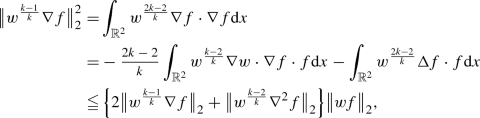(B.1)

where we have used the assumption (3.5) that $$1\leqq w$$ and $$|\nabla w|\leqq w$$. Since $$w^{\frac{k-1}{k}}$$ as a weight also satisfies the assumption (3.5), by induction we have

\begin{aligned}\begin{aligned} \big \Vert w^{\frac{k-2}{k}}\nabla ^2f\big \Vert _{2} =&\big \Vert (w^{\frac{k-1}{k}})^{\frac{(k-1)-1}{(k-1)}} \nabla (\nabla f)\big \Vert _{2} \\ \leqq&\,4^{k-1}\Big \{\big \Vert w^{\frac{k-1}{k}}\nabla f\big \Vert _{2} +\big \Vert \nabla ^{k-1}(\nabla f)\big \Vert _{2}\Big \}. \end{aligned} \end{aligned}

Therefore, from (B.1), we have

\begin{aligned} \begin{aligned} \big \Vert w^{\frac{k-1}{k}}\nabla f\big \Vert _{2}^2 \leqq&(4^{k-1}+2)\Big \{\big \Vert w^{\frac{k-1}{k}}\nabla f\big \Vert _{2} +\big \Vert \nabla ^k f\big \Vert _{2}\Big \}\big \Vert wf\big \Vert _{2} \\ \leqq&\, \frac{1}{2}\big \Vert w^{\frac{k-1}{k}}\nabla f\big \Vert _{2}^2 +2(4^{k-1}+2)^2\Big \{\big \Vert wf\big \Vert _{2}^2+\big \Vert \nabla ^k f\big \Vert _{2}^2\Big \}, \end{aligned} \end{aligned}

which proves (3.6) for $${\bar{k}}=k$$ and $${\bar{j}}=1$$ since $$\sqrt{4(4^{k-1}+2)^2}\leqq 4^k$$ when $$k\geqq 2$$.

Fix $${\bar{k}}=k$$. Let j be an integer such that $$2\leqq j\leqq k$$, suppose that we have proved (3.6) for all $${\bar{j}}$$ satisfying $$0\leqq {\bar{j}}\leqq j-1$$. Now we shall prove (3.6) for $${\bar{k}}=k$$ and $${\bar{j}}=j$$.

We still use the fact that $$w^{\frac{k-1}{k}}$$ as a weight also satisfies the assumption (3.5). By induction, it holds that

\begin{aligned} \begin{aligned} \big \Vert w^{\frac{k-j}{k}}\nabla ^j f\big \Vert _{2}&= \big \Vert (w^{\frac{k-1}{k}})^{\frac{(k-1)-(j-1)}{(k-1)}} \nabla ^{j-1}(\nabla f)\big \Vert _{2} \\&\leqq \, 4^{(k-1)(j-1)}\Big \{\big \Vert w^{\frac{k-1}{k}}\nabla f\big \Vert _{2} +\big \Vert \nabla ^{k-1}(\nabla f)\big \Vert _{2}\Big \}\\&\leqq \, 4^{(k-1)(j-1)}\Big \{4^k\big \{\big \Vert wf\big \Vert _{2}+\big \Vert \nabla ^k f\big \Vert _{2}\big \}+\big \Vert \nabla ^k f\big \Vert _{2}\Big \}\\&\leqq \, 4^{(k-1)(j-1)}\cdot 4^k\cdot 2 \Big \{\big \Vert wf\big \Vert _{2}+\big \Vert \nabla ^k f\big \Vert _{2}\Big \}, \end{aligned} \end{aligned}

which proves (3.6) since $$4^{(k-1)(j-1)}\cdot 4^k\cdot 2\leqq 4^{k\cdot j}$$ when $$j\geqq 2$$.

To prove (3.7), noticing that $$|\nabla w|\leqq w$$ and $$H^{2}({\mathbb {R}}^2)\subset L^{\infty }({\mathbb {R}}^2)$$, we have

\begin{aligned} \begin{aligned} \big \Vert w^{\frac{k-j}{k}}\nabla ^{j-2} f\big \Vert _{\infty } \leqq&C_s\big \Vert \nabla ^{\leqq 2}\big (w^{\frac{k-j}{k}}\nabla ^{j-2} f\big )\big \Vert _{2} \\&\leqq \, 4C_s\big \Vert w^{\frac{k-j}{k}}\nabla ^{\leqq j} f\big \Vert _{2} \\&\leqq \, C_s\cdot 4^{k\cdot j+1}\Big \{\big \Vert wf\big \Vert _{2}+\big \Vert \nabla ^k f\big \Vert _{2}\Big \}. \end{aligned} \end{aligned}

### Appendix C. Estimates of Some Commutators

In this appendix, we obtain some estimates for the following commutators:

\begin{aligned} {[}\nabla \Delta ^{-1}\nabla \cdot ,\,v\cdot \nabla ]w=\nabla \Delta ^{-1}\nabla \cdot (v\cdot \nabla w)-v\cdot \nabla (\nabla \Delta ^{-1}\nabla \cdot w), \end{aligned}

and

\begin{aligned} {[}\nabla ^{\perp }\Delta ^{-1}\nabla ^{\perp }\cdot ,\,v\cdot \nabla ]w=\nabla ^{\perp }\Delta ^{-1}\nabla ^{\perp }\cdot (v\cdot \nabla w)-v\cdot \nabla (\nabla ^{\perp }\Delta ^{-1}\nabla ^{\perp }\cdot w). \end{aligned}

### Lemma C.1

Assume that vectors $$v\in W^{1,\infty }({\mathbb {R}}^2)$$, $$w\in H^{1}({\mathbb {R}}^2)$$ and v, w decay fast enough at infinity. Then

\begin{aligned} \big \Vert [\nabla \Delta ^{-1}\nabla \cdot ,\,v\cdot \nabla ]w\big \Vert _{2} +\big \Vert [\nabla ^{\perp }\Delta ^{-1}\nabla ^{\perp }\cdot ,\,v\cdot \nabla ]w\big \Vert _{2} \lesssim \big \Vert \nabla v\big \Vert _{\infty }\big \Vert w\big \Vert _{2}. \end{aligned}
(C.1)

Moreover if functions $$b\in W^{1,\infty }({\mathbb {R}}^2)$$, $$c\in H^1({\mathbb {R}}^2)$$ and b, c decay fast enough at infinity, then

\begin{aligned} \big \Vert \nabla \Delta ^{-1}\nabla \cdot (b\nabla c)-b\nabla c\big \Vert _{2} =\big \Vert \nabla ^{\perp }\Delta ^{-1}\nabla ^{\perp }\cdot (b\nabla c)\big \Vert _{2} \lesssim \big \Vert \nabla b\big \Vert _{\infty }\big \Vert c\big \Vert _{2}. \end{aligned}
(C.2)

### Proof

Choose $$\phi \in C_0^{\infty }({\mathbb {R}}^2)$$ and denote the Hodge decompositions of $$\phi$$ and w by

\begin{aligned} \phi= & {} \nabla \Delta ^{-1}\nabla \cdot \phi +\nabla ^{\perp }\Delta ^{-1}\nabla ^{\perp }\cdot \phi =\phi _{\mathrm {com}}+\phi _{\mathrm {in}}, \\ w= & {} \nabla \Delta ^{-1}\nabla \cdot w+\nabla ^{\perp }\Delta ^{-1}\nabla ^{\perp }\cdot w=w_{\mathrm {com}}+w_{\mathrm {in}}. \end{aligned}

Then

\begin{aligned} \begin{aligned}&\int _{{\mathbb {R}}^2}\phi \cdot [\nabla \Delta ^{-1}\nabla \cdot ,\,v\cdot \nabla ]w \text {d}x \\&\quad = \int _{{\mathbb {R}}^2}\phi \cdot \Big \{\nabla \Delta ^{-1}\nabla \cdot (v\cdot \nabla w)\Big \}\text {d}x -\int _{{\mathbb {R}}^2}\phi \cdot \Big \{v\cdot \nabla (\nabla \Delta ^{-1}\nabla \cdot w)\Big \}\text {d}x \\&\quad = \int _{{\mathbb {R}}^2}\phi _{\mathrm {com}}\cdot (v\cdot \nabla w)\text {d}x -\int _{{\mathbb {R}}^2}\phi \cdot (v\cdot \nabla w_{\mathrm {com}}) \text {d}x \\&\quad = \int _{{\mathbb {R}}^2}\phi _{\mathrm {com}}\cdot (v\cdot \nabla w_{\mathrm {in}})\text {d}x -\int _{{\mathbb {R}}^2}\phi _{\mathrm {in}}\cdot (v\cdot \nabla w_{\mathrm {com}}) \text {d}x \\&\quad = -\,\int _{{\mathbb {R}}^2}(\nabla \cdot v) (w_{\mathrm {in}}\cdot \phi _{\mathrm {com}}) \text {d}x -\int _{{\mathbb {R}}^2}w_{\mathrm {in}}\cdot (v\cdot \nabla \phi _{\mathrm {com}}) \text {d}x -\int _{{\mathbb {R}}^2}\phi _{\mathrm {in}}\cdot (v\cdot \nabla w_{\mathrm {com}}) \text {d}x. \end{aligned} \end{aligned}
(C.3)

Since $$\nabla _k(\phi _{\mathrm {com}})^i=\nabla _i(\phi _{\mathrm {com}})^k$$ and $$w_{\mathrm {in}}$$ is divergence-free, the second term on the right hand side of (C.3) is

\begin{aligned} \begin{aligned}&\int _{{\mathbb {R}}^2}w_{\mathrm {in}}\cdot (v\cdot \nabla \phi _{\mathrm {com}}) \text {d}x = \int _{{\mathbb {R}}^2}(w_{\mathrm {in}})^i v^k\nabla _k(\phi _{\mathrm {com}})^i \text {d}x \\&\quad = \int _{{\mathbb {R}}^2}(w_{\mathrm {in}})^i v^k\nabla _i(\phi _{\mathrm {com}})^k \text {d}x = -\,\int _{{\mathbb {R}}^2}(\nabla _i v^k) (w_{\mathrm {in}})^i(\phi _{\mathrm {com}})^k \text {d}x. \end{aligned} \end{aligned}

A similar result holds true for the last term on the right hand side of (C.3) if we exchange the positions of w and $$\phi$$. Therefore, we have

\begin{aligned} \Big |\int _{{\mathbb {R}}^2}\phi \cdot [\nabla \Delta ^{-1}\nabla \cdot ,\,v\cdot \nabla ]w \text {d}x\Big | \lesssim \big \Vert \nabla v\big \Vert _{\infty }\big \Vert w\big \Vert _{2}\big \Vert \phi \big \Vert _{2}. \end{aligned}

This yields the estimate of the first term on the left hand side of (C.1). For the second term,

\begin{aligned}\begin{aligned}&\int _{{\mathbb {R}}^2}\phi \cdot [\nabla ^{\perp }\Delta ^{-1}\nabla ^{\perp }\cdot ,\,v\cdot \nabla ]w \text {d}x \\&\quad = \int _{{\mathbb {R}}^2}\phi \cdot \Big \{\nabla ^{\perp }\Delta ^{-1}\nabla ^{\perp }\cdot (v\cdot \nabla w)\Big \}\text {d}x -\int _{{\mathbb {R}}^2}\phi \cdot \Big \{v\cdot \nabla (\nabla ^{\perp }\Delta ^{-1}\nabla ^{\perp }\cdot w)\Big \}\text {d}x \\&\quad = \int _{{\mathbb {R}}^2}\phi _{\mathrm {in}}\cdot (v\cdot \nabla w) \text {d}x -\int _{{\mathbb {R}}^2}\phi \cdot (v\cdot \nabla w_{\mathrm {in}}) \text {d}x \\&\quad = \int _{{\mathbb {R}}^2}\phi _{\mathrm {in}}\cdot (v\cdot \nabla w_{\mathrm {com}}) \text {d}x -\int _{{\mathbb {R}}^2}\phi _{\mathrm {com}}\cdot (v\cdot \nabla w_{\mathrm {in}}) \text {d}x \\&\quad = -\,\int _{{\mathbb {R}}^2}\phi \cdot [\nabla \Delta ^{-1}\nabla \cdot ,\,v\cdot \nabla ]w \text {d}x, \end{aligned} \end{aligned}

and hence

\begin{aligned} \Big |\int _{{\mathbb {R}}^2}\phi \cdot [\nabla ^{\perp }\Delta ^{-1}\nabla ^{\perp }\cdot ,\,v\cdot \nabla ]w \text {d}x\Big | \lesssim \big \Vert \nabla v\big \Vert _{\infty }\big \Vert w\big \Vert _{2}\big \Vert \phi \big \Vert _{2}. \end{aligned}

This proves (C.1).

For (C.2), since $$\phi _{\mathrm {in}}$$ is divergence-free,

\begin{aligned}\begin{aligned}&\int _{{\mathbb {R}}^2}\phi \cdot \Big \{\nabla \Delta ^{-1}\nabla \cdot (b\nabla c)\Big \}\text {d}x -\int _{{\mathbb {R}}^2}\phi \cdot (b\nabla c)\text {d}x \\&\quad = \int _{{\mathbb {R}}^2}\phi _{\mathrm {com}}\cdot (b\nabla c)\text {d}x -\int _{{\mathbb {R}}^2}\phi \cdot (b\nabla c)\text {d}x \\&\quad = -\,\int _{{\mathbb {R}}^2}\phi _{\mathrm {in}}\cdot (b\nabla c)\text {d}x \\&\quad = \int _{{\mathbb {R}}^2}c (\phi _{\mathrm {in}}\cdot \nabla b)\text {d}x, \end{aligned} \end{aligned}

which means that

\begin{aligned} \Big |\int _{{\mathbb {R}}^2}\phi \cdot \Big \{\nabla \Delta ^{-1}\nabla \cdot (b\nabla c)\Big \}\text {d}x -\int _{{\mathbb {R}}^2}\phi \cdot (b\nabla c)\text {d}x\Big | \lesssim \big \Vert \nabla b\big \Vert _{\infty }\big \Vert c\big \Vert _{2}\big \Vert \phi \big \Vert _{2}. \end{aligned}

Moreover,

\begin{aligned} \begin{aligned}&\Big |\int _{{\mathbb {R}}^2}\phi \cdot \Big \{\nabla ^{\perp }\Delta ^{-1}\nabla ^{\perp }\cdot (b\nabla c) \Big \}\text {d}x\Big | \\&\quad = \Big |\int _{{\mathbb {R}}^2}\phi _{\mathrm {in}}\cdot (b\nabla c)\text {d}x\Big | \\&\quad = \Big |-\int _{{\mathbb {R}}^2}\phi \cdot \Big \{\nabla \Delta ^{-1}\nabla \cdot (b\nabla c)\Big \}\text {d}x -\int _{{\mathbb {R}}^2}\phi \cdot (b\nabla c)\text {d}x\Big | \\&\quad \lesssim \big \Vert \nabla b\big \Vert _{\infty }\big \Vert c\big \Vert _{2}\big \Vert \phi \big \Vert _{2}, \end{aligned} \end{aligned}

and which verifies (C.2). $$\quad \square$$

### Appendix D. The Green’s Function for Compressible Parts

In this appendix, we shall study the Green’s function for the compressible system (2.11). For $$\alpha =(\alpha _s,\,\alpha _z)$$, since $$\Phi ^{\alpha }$$ can be recovered by the constraint (2.12), we just need to solve the following linear system for the compressible parts $$(\eta ^{\alpha },\,\varphi ^{\alpha })\in {\mathbb {R}}^2\times {\mathbb {R}}$$ with suitable initial data and zero source terms:

\begin{aligned} {\left\{ \begin{array}{ll} \partial _t\eta ^{\alpha }+\nabla \varphi ^{\alpha }=0, \\ \partial _t\varphi ^{\alpha }+2\nabla \cdot \eta ^{\alpha }+\mathop {\sum }\limits _{j=0}^{\alpha _s}(-1)^{\alpha _s-j}\left( {\begin{array}{c}\alpha _s\\ j\end{array}}\right) \Delta \varphi ^{(j,\,\alpha _z)}=0, \\ \nabla ^{\perp }\cdot \eta ^{\alpha }=0, \end{array}\right. } \end{aligned}
(D.1)

where we have kept the binomial coefficients $$\left( {\begin{array}{c}\alpha _s\\ j\end{array}}\right)$$.

The process will be separated into several steps.

### D.1. The Green’s Function for $$(\eta ,\,\varphi )$$

Let $$\alpha =0$$ and consider the following system for functions $$(\eta ,\,\varphi )\in {\mathbb {R}}^2\times {\mathbb {R}}$$:

\begin{aligned} {\left\{ \begin{array}{ll} \partial _t\eta +\nabla \varphi =0, \\ \partial _t\varphi +2\nabla \cdot \eta -\Delta \varphi =0, \\ \nabla ^{\perp }\cdot \eta =0. \end{array}\right. } \end{aligned}
(D.2)

Taking the Fourier transform in space gives

\begin{aligned} \partial _t\begin{pmatrix}{\widehat{\eta }}\\ {\widehat{\varphi }}\end{pmatrix} =\begin{pmatrix}0_{2\times 2} &{} -\sqrt{-1}\xi \\ -2\sqrt{-1}\xi ^{\top }&{} -|\xi |^2\end{pmatrix} \begin{pmatrix}{\widehat{\eta }}\\ {\widehat{\varphi }}\end{pmatrix}. \end{aligned}

The eigenvalues for the matrix are

\begin{aligned} \lambda _0=0,\quad \quad \lambda _{\pm }=-\,\frac{1}{2}|\xi |^2\pm \frac{1}{2}\sqrt{|\xi |^4-8|\xi |^2}, \end{aligned}
(D.3)

and the Green’s function is given by

\begin{aligned} G(t,\,x)={\mathfrak {F}}^{-1}\begin{pmatrix} \frac{\lambda _+ e^{\lambda _-t}-\lambda _- e^{\lambda _+t}}{\lambda _+-\lambda _-} I_{2\times 2} &{} -\sqrt{-1}\frac{e^{\lambda _+t}-e^{\lambda _-t}}{\lambda _+-\lambda _-}\xi \\ -2\sqrt{-1}\frac{e^{\lambda _+t}-e^{\lambda _-t}}{\lambda _+-\lambda _-}\xi ^{\top }&{} \frac{\lambda _+ e^{\lambda _+t}-\lambda _- e^{\lambda _-t}}{\lambda _+-\lambda _-} \\ \end{pmatrix}, \end{aligned}
(D.4)

where we have used the constraint in (D.2). Since(D.5)

we have for $$|\xi |\ll 1$$ that

\begin{aligned} {\widehat{G}}(t,\,\xi )\sim e^{-\frac{|\xi |^2}{2}t} \begin{pmatrix} \cos (\sqrt{2}|\xi |t) I_{2\times 2} &{} -\frac{\sqrt{-2}}{2}\sin (\sqrt{2}|\xi |t)\frac{\xi }{|\xi |} \\ -\sqrt{-2}\sin (\sqrt{2}|\xi |t) \frac{\xi ^{\top }}{|\xi |} &{} \cos (\sqrt{2}|\xi |t) \\ \end{pmatrix} + O(|\xi |), \end{aligned}

while

\begin{aligned} {\left\{ \begin{array}{ll} \lambda _+\sim -2+O(|\xi |^{-2}), \\ \lambda _-\sim -|\xi |^2+2+O(|\xi |^{-2}), \\ (\lambda _+-\lambda _-)\sim |\xi |^2-4+O(|\xi |^{-2}), \end{array}\right. } \text { for }|\xi |\gg 1, \end{aligned}
(D.6)

hence, for $$|\xi |\gg 1$$,

\begin{aligned} {\widehat{G}}(t,\,\xi )\sim e^{-2t} \begin{pmatrix} I_{2\times 2} &{} -\sqrt{-1}\frac{\xi }{|\xi |^2} \\ -2\sqrt{-1}\frac{\xi ^{\top }}{|\xi |^2} &{} 0 \\ \end{pmatrix}+O(|\xi |^{-2}). \end{aligned}

### Lemma D.1

For $$i\geqq 1$$ and $$1\leqq q\leqq 2 \leqq p \leqq \infty$$, if holds that

\begin{aligned} \begin{aligned}&\big \Vert \nabla ^i G(t,\,\cdot ) *(\eta ,\,\varphi )^{\top }(0,\,\cdot )\big \Vert _p \\&\quad \lesssim \langle t\rangle ^{-\left( \frac{1}{q}-\frac{1}{p}+\frac{i}{2}\right) } \Big \{\big \Vert (\eta ,\,\varphi )_{t=0}\big \Vert _q +\big \Vert (\nabla ^i\eta ,\,\nabla ^i\varphi )_{t=0}\big \Vert _p\Big \}. \end{aligned} \end{aligned}

The proof can be found in [9, 13, 23, 27] for similar systems.

### D.2. The Green’s Function for $$(S^k\eta ,\,S^k\varphi )$$

Let $$\alpha =(\alpha _s,\,\alpha _z)$$ with $$\alpha _s=k$$ and $$\alpha _z=0$$ in (D.1). Also consider the linear system for $$(\eta ^k,\,\varphi ^k)=(S^k\eta ,\,S^k\varphi )$$

\begin{aligned} {\left\{ \begin{array}{ll} \partial _t\eta ^k+\nabla \varphi ^k=0, \\ \partial _t\varphi ^k+2\nabla \cdot \eta ^k-\Delta \varphi ^k =\mathop {\sum }\limits _{l=0}^{k-1}(-1)^{k-l}\left( {\begin{array}{c}k\\ l\end{array}}\right) \Delta \varphi ^l, \\ \nabla ^{\perp }\cdot \eta ^k=0. \end{array}\right. } \end{aligned}
(D.7)

The Fourier transform for the first two equations in (D.7) gives the following equation for $${\widehat{\varphi }}^k$$:

\begin{aligned} \partial _t^2{\widehat{\varphi }}^k+|\xi |^2\partial _t{\widehat{\varphi }}^k+2|\xi |^2{\widehat{\varphi }}^k =-\,\mathop {\sum }\limits _{l=0}^{k-1}(-1)^{k-l}\left( {\begin{array}{c}k\\ l\end{array}}\right) |\xi |^2\partial _t{\widehat{\varphi }}^l. \end{aligned}
(D.8)

We shall use induction to solve $$\{{\widehat{\varphi }}^l\}_{l=0}^k$$. Assume that the solutions $${\widehat{\varphi }}^l$$$$(0\leqq l\leqq k)$$ are given by

\begin{aligned} {\widehat{\varphi }}^l=\mathop {\sum }\limits _{j=0}^lA^l_j t^je^{\lambda _+t}+\mathop {\sum }\limits _{j=0}^lB^l_jt^je^{\lambda _-t}, \quad \quad 0\leqq l\leqq k, \end{aligned}

with $$A^l_j=A^l_j(\xi )$$, $$B^l_j=B^l_j(\xi )$$ and $$\lambda _{\pm }$$ defined in (D.3). Thus the equation can be rewritten in terms of the quasi-exponentials $$t^je^{\lambda _{\pm }t}$$:

\begin{aligned} \begin{aligned}&\mathop {\sum }\limits _{j=0}^{k-1}\Big \{(j+1)A^k_{j+1}(\lambda _+-\lambda _-)\Big \}t^je^{\lambda _+t} +\mathop {\sum }\limits _{j=0}^{k-2}\Big \{(j+2)(j+1)A^k_{j+2}\Big \}t^je^{\lambda _+t} \\&\qquad -\mathop {\sum }\limits _{j=0}^{k-1}\Big \{(j+1)B^k_{j+1}(\lambda _+-\lambda _-)\Big \}t^je^{\lambda _-t} +\mathop {\sum }\limits _{j=0}^{k-2}\Big \{(j+2)(j+1)B^k_{j+2}\Big \}t^je^{\lambda _-t} \\&\quad = -\,\mathop {\sum }\limits _{j=0}^{k-1} \Big \{\mathop {\sum }\limits _{l=j}^{k-1}(-1)^{k-l}\left( {\begin{array}{c}k\\ l\end{array}}\right) \lambda _+|\xi |^2A^l_j\Big \}t^je^{\lambda _+t} \\&\qquad -\,\mathop {\sum }\limits _{j=0}^{k-2} \Big \{\mathop {\sum }\limits _{l=j+1}^{k-1}(-1)^{k-l}\left( {\begin{array}{c}k\\ l\end{array}}\right) (j+1)A^l_{j+1}|\xi |^2\Big \}t^je^{\lambda _+t} \\&\qquad -\,\mathop {\sum }\limits _{j=0}^{k-1} \Big \{\mathop {\sum }\limits _{l=j}^{k-1}(-1)^{k-l}\left( {\begin{array}{c}k\\ l\end{array}}\right) \lambda _+|\xi |^2B^l_j\Big \}t^je^{\lambda _-t} \\&\qquad -\,\mathop {\sum }\limits _{j=0}^{k-2} \Big \{\mathop {\sum }\limits _{l=j+1}^{k-1}(-1)^{k-l}\left( {\begin{array}{c}k\\ l\end{array}}\right) (j+1)B^l_{j+1}|\xi |^2\Big \}t^je^{\lambda _-t}. \end{aligned} \end{aligned}

Compare the coefficients of the quasi-exponentials $$t^je^{\lambda _{\pm }t}$$ in the equation above to get that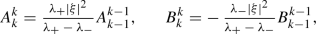(D.9)

and for $$1\leqq j\leqq k-1$$,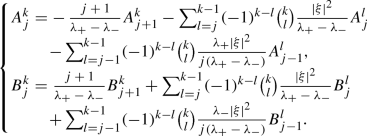(D.10)

For $$j=0$$, the initial data $$({\widehat{\varphi }}^k,\,\partial _t{\widehat{\varphi }}^k)|_{t=0}$$ gives(D.11)

Then we can prove the following estimates:

### Lemma D.2

For all $$0\leqq j\leqq k$$, we have

\begin{aligned} |\xi |^{-2j}|A^k_j|+|\xi |^{-2j}|B^k_j|\lesssim \mathop {\sum }\limits _{l=0}^{k}\big \vert ({\widehat{\eta }}^l,\,{\widehat{\varphi }}^l)_{t=0}\big \vert , \quad \quad \text { for }|\xi |\ll 1, \end{aligned}
(D.12)

and

\begin{aligned} |A^k_j|+|\xi |^{-2j}|B^k_j| \lesssim \mathop {\sum }\limits _{l=0}^{k} \big \vert ({\widehat{\eta }}^l,\,{\widehat{\varphi }}^l)_{t=0}\big \vert , \quad \text { for }|\xi |\gg 1. \end{aligned}
(D.13)

### Proof

For $$A^0_0$$ and $$B^0_0$$, the Green’s function in (D.4) implies thatThus the estimates for $$A^0_0$$ and $$B^0_0$$ in the lemma follow from (D.5) and (D.6).

For $$A^k_k$$ and $$B^k_k$$, an induction of (D.9) shows thatandwhere we have used (D.5) and (D.6).

Now suppose that we have proved (D.12) and (D.13) for all $$A^{{\bar{k}}}_{{\bar{j}}}$$ and $$B^{{\bar{k}}}_{{\bar{j}}}$$ with $$\{({\bar{k}},\,{\bar{j}}): 0\leqq {\bar{j}}\leqq {\bar{k}}\leqq k-1\}$$ and $$\{({\bar{k}},\,{\bar{j}}):{\bar{k}}=k,\,j+1\leqq {\bar{j}}\leqq k\}$$; see Fig. 1 for an illustration of the process. We shall prove that $$A^k_j$$ and $$B^k_j$$ with $$j\geqq 1$$ also satisfy the estimates in (D.12) and (D.13). From (D.10) and (D.5), (D.6), it is easy to check that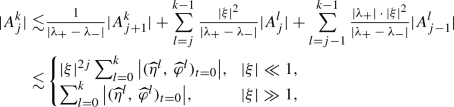and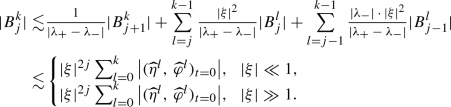Finally, for $$A^k_0$$ and $$B^k_0$$ given by (D.11), since the Fourier transform of the second equation in (D.7) yields

\begin{aligned} \partial _t{\widehat{\varphi }}^k=-\,2\sqrt{-1}\xi \cdot {\widehat{\eta }}^k -|\xi |^2{\widehat{\varphi }}^k -\mathop {\sum }\limits _{l=0}^{k-1}(-1)^{k-l}\left( {\begin{array}{c}k\\ l\end{array}}\right) |\xi |^2{\widehat{\varphi }}^l, \end{aligned}

we haveandThus we have proved the lemma. $$\quad \square$$

Now we can derive the estimates for the solution $$({\widehat{\eta }}^k,\,{\widehat{\varphi }}^k)$$ when $$t>0$$ is large. From the estimates of $$A^k_j$$ and $$B^k_j$$ in (D.12) and (D.13),

\begin{aligned} \begin{aligned} |{\widehat{\varphi }}^k|\leqq&\mathop {\sum }\limits _{j=0}^k |A^k_j| t^je^{\lambda _+t} +\mathop {\sum }\limits _{j=0}^k |B^k_j| t^je^{\lambda _-t} \\ \lesssim&{\left\{ \begin{array}{ll} \mathop {\sum }\limits _{j=0}^k(|\xi |^2t)^j e^{-\frac{|\xi |^2}{2}t} \mathop {\sum }\limits _{l=0}^{k}\big \vert ({\widehat{\eta }}^l,\,{\widehat{\varphi }}^l)_{t=0}\big \vert , &{} |\xi |\ll 1, \\ \mathop {\sum }\limits _{j=0}^k t^je^{-2t} \mathop {\sum }\limits _{l=0}^{k}\big \vert ({\widehat{\eta }}^l,\,{\widehat{\varphi }}^l)_{t=0}\big \vert , &{} |\xi |\gg 1, \end{array}\right. } \\ \lesssim&{\left\{ \begin{array}{ll} e^{-\frac{|\xi |^2}{4}t} \mathop {\sum }\limits _{l=0}^{k}\big \vert ({\widehat{\eta }}^l,\,{\widehat{\varphi }}^l)_{t=0}\big \vert , &{} |\xi |\ll 1, \\ e^{-t} \mathop {\sum }\limits _{l=0}^{k}\big \vert ({\widehat{\eta }}^l,\,{\widehat{\varphi }}^l)_{t=0}\big \vert , &{} |\xi |\gg 1. \end{array}\right. } \end{aligned} \end{aligned}
(D.14)

On the other hand, for $${\widehat{\eta }}^k$$, the Fourier transform of the last two equations in (D.7) shows that

\begin{aligned} \begin{aligned} {\widehat{\eta }}^k&= \sqrt{-1}\frac{\xi }{2|\xi |^2}\Big \{\partial _t{\widehat{\varphi }}^k +\mathop {\sum }\limits _{l=0}^{k}(-1)^{k-l}\left( {\begin{array}{c}k\\ l\end{array}}\right) |\xi |^2{\widehat{\varphi }}^l\Big \}\\&= \sqrt{-1}\frac{\xi }{2|\xi |^2}\mathop {\sum }\limits _{j=1}^k \Big \{jA^k_j t^{j-1}e^{\lambda _+t}+jB^k_j t^{j-1}e^{\lambda _-t}\Big \}\\&\quad +\,\sqrt{-1}\frac{\xi }{2|\xi |^2}\mathop {\sum }\limits _{j=0}^k \Big \{\lambda _+A^k_j t^je^{\lambda _+t}+\lambda _-B^k_jt^je^{\lambda _-t}\Big \}\\&\quad +\,\sqrt{-1}\frac{\xi }{2|\xi |^2}\mathop {\sum }\limits _{l=0}^k(-1)^{k-l}\left( {\begin{array}{c}k\\ l\end{array}}\right) |\xi |^2\mathop {\sum }\limits _{j=0}^l\Big \{A^l_j t^j e^{\lambda _+t} +B^l_j t^j e^{\lambda _-t}\Big \}. \end{aligned} \end{aligned}

As a result, we can apply (D.5), (D.6) and (D.12), (D.13) to get that

\begin{aligned} \begin{aligned} |{\widehat{\eta }}^k|\lesssim&{\left\{ \begin{array}{ll} \mathop {\sum }\limits _{j=0}^k(|\xi |^2t)^j e^{-\frac{|\xi |^2}{2}t} \mathop {\sum }\limits _{l=0}^{k}\big \vert ({\widehat{\eta }}^l,\,{\widehat{\varphi }}^l)_{t=0}\big \vert , &{} |\xi |\ll 1, \\ \mathop {\sum }\limits _{j=0}^k t^je^{-2t} \mathop {\sum }\limits _{l=0}^{k}\big \vert (|\xi |{\widehat{\eta }}^l,\,|\xi |{\widehat{\varphi }}^l)_{t=0}\big \vert , &{} |\xi |\gg 1, \end{array}\right. } \\ \lesssim&{\left\{ \begin{array}{ll} e^{-\frac{|\xi |^2}{4}t} \mathop {\sum }\limits _{l=0}^{k}\big \vert ({\widehat{\eta }}^l,\,{\widehat{\varphi }}^l)_{t=0}\big \vert , &{} |\xi |\ll 1, \\ e^{-t}\mathop {\sum }\limits _{l=0}^{k}\big \vert (|\xi |{\widehat{\eta }}^l,\,|\xi |{\widehat{\varphi }}^l)_{t=0}\big \vert , &{} |\xi |\gg 1. \end{array}\right. } \end{aligned} \end{aligned}
(D.15)

Instead of the system (D.7) for $$(\eta ^k,\,\varphi ^k)$$, we shall consider a larger system for all $$(\eta ^j,\,\varphi ^j)_{j=0}^k$$. Denote the Green’s function for the larger system by G again. Then from [9, 13, 23, 27], (D.15) and (D.14) imply the following decay estimates:

### Lemma D.3

For $$i\geqq 1$$ and $$1\leqq q\leqq 2 \leqq p \leqq \infty$$, it holds that

\begin{aligned} \begin{aligned}&\big \Vert \nabla ^i G(t,\,\cdot ) *\big \{(\eta ^j,\,\varphi ^j)^{\top }(0,\,\cdot )\big \}_{j=0}^k\big \Vert _p \\&\quad \lesssim \langle t\rangle ^{-\left( \frac{1}{q}-\frac{1}{p}+\frac{i}{2}\right) } \mathop {\sum }\limits _{j=0}^k\Big \{\big \Vert (\eta ^j,\,\varphi ^j)_{t=0}\big \Vert _q +\big \Vert (\nabla ^{i+1}\eta ^j,\,\nabla ^{i+1}\varphi ^j)_{t=0}\big \Vert _p\Big \}. \end{aligned} \end{aligned}

Here we should notice that the order of regularity required for the initial data is $$(i+1)$$ in Lemma D.3 instead of i in Lemma D.1. This is due to the fact that, in (D.15) when $$|\xi |\gg 1$$, we need one more regularity for the initial data.

### D.3. The Green’s Function for $$(\eta ^{\alpha },\,\varphi ^{\alpha })$$

For general $$\alpha =(\alpha _s,\,\alpha _z)$$, the vector fields $$Z=\{{\widetilde{\Omega }},\,\nabla \}$$ commute with the system (D.1) very well. When solving $$({\widehat{\eta }}^{(k,\,\alpha _z)},\,{\widehat{\varphi }}^{(k,\,\alpha _z)})$$ for $$0\leqq k\leqq \alpha _s$$, we have a similar form of solution as to those $$({\widehat{\eta }}^k,\,{\widehat{\varphi }}^k)$$ using quasi-exponentials (D.9)–(D.11), and the decay estimates are the same as those in Lemma D.3, except that now we need to consider an even larger system. As a result, when deriving the decay estimates of the compressible parts in (2.11), we can just consider the case $$\nabla \cdot {\mathcal {L}}_{c}^{\alpha }=0$$ and focus on each system for $$(\eta ^{\alpha },\,\varphi ^{\alpha })$$ to get

\begin{aligned} {\left\{ \begin{array}{ll} \partial _t\eta ^{\alpha }+\nabla \varphi ^{\alpha }=0, \\ \partial _t\varphi ^{\alpha }+2\nabla \cdot \eta ^{\alpha }-\Delta \varphi ^{\alpha }={\mathcal {N}}^{\alpha }, \\ \nabla ^{\perp }\cdot \eta ^{\alpha }=0. \end{array}\right. } \end{aligned}

We even abuse the use of the Green’s function G further, so that the solution is given explicitly by

\begin{aligned} \begin{pmatrix} \eta ^{\alpha }\\ \varphi ^{\alpha }\end{pmatrix} =G(t,\,\cdot )*\begin{pmatrix} \eta ^{\alpha }\\ \varphi ^{\alpha }\end{pmatrix}(0,\,\cdot ) +\int _{0}^{t}G(t-s,\,\cdot )*{\mathcal {N}}^{\alpha }(s,\,\cdot )\text {d}s, \end{aligned}

where we should have used $$(0,\,{\mathcal {N}}^{\alpha })^{\top }$$ as the nonlinear term.

Then the Green’s function satisfies the following:

### Lemma D.4

For $$i\geqq 1$$ and $$1\leqq q\leqq 2 \leqq p \leqq \infty$$, it holds that

\begin{aligned} \begin{aligned}&\big \Vert \nabla ^i G(t,\,\cdot ) *(\eta ^{\alpha },\,\varphi ^{\alpha })^{\top }(0,\,\cdot )\big \Vert _p \\&\quad \lesssim \langle t\rangle ^{-\left( \frac{1}{q}-\frac{1}{p}+\frac{i}{2}\right) } \Big \{\big \Vert (\eta ^{\alpha },\,\varphi ^{\alpha })_{t=0}\big \Vert _q +\big \Vert (\nabla ^{i+1}\eta ^{\alpha },\,\nabla ^{i+1}\varphi ^{\alpha })_{t=0}\big \Vert _p\Big \}. \end{aligned} \end{aligned}
(D.16)

## Rights and permissions

Reprints and Permissions